Points, lines and circles associated with a triangle

Sandra Fink

E-mail: sandra.fink@edu.uni-graz.at
Steckbrief

 Übersicht:Hilfe
1. Elementary Geometry Revisited
2. Euler Line
3. Nine-Point-Circle
 Elementary Geometry Revisited
 1.1 Back to the roots...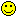The purpose of this "Lernpfad" is to give a brief insight into some of the most important and interesting theorems of Euclidean Geometry, especially into the geometry of triangles. Before you go on the journey, make sure that you repeat the basics about triangles. This summary (click on the word "summary"!) may help you with this! In order NOT to cause too much needless confusion I will stick to the following nomenclature: Common Nomenclature Have fun :-) take notes in your portfolio, sketch triangles
 1.2 Altitudes of a triangle & the orthocenter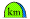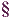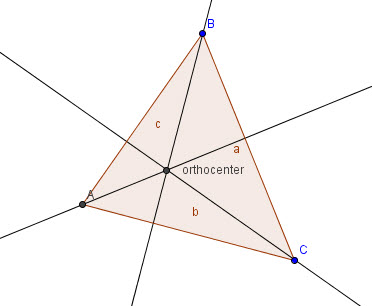Short repetition: Draw any triangle ABC and the altitudes of the triangle with GeoGebra. What do you notice? Don't forget to save your file! Definition: In Geometry, the altitudes of a triangle are the straight lines through a vertex and which is perpenticular to (i.e. forming a right angle with) the opposite side or an extension of the opposite side. In other words, it starts at a vertex and is perpendicular to the opposite side Furthermore, the three altitudes intersect in a single point, called the orthocenter of the triangle. take notes in your portfolio, repetition
 1.3 Exercise - altitudes & the orthocenterClick on Assignment 1 to get your first assignment. exercise
 1.4 Experiment - Can you balance a triangle???Imagine you have a triangular metal plate (or a triangular set square), and try to balance it on a point - say a pencil tip. How can you do that? Is there a special point at which it will balace? Why don't you try it out with your set square and a pencil??1.5 Medians of a triangle & the centroid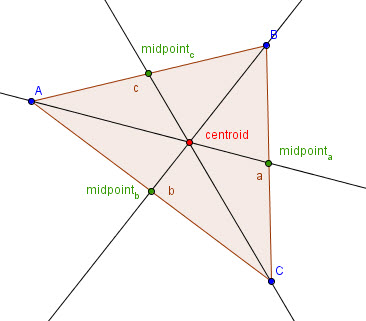Definition: In Geometry, a median of a triangle is a line segment joining a vertex to the midpoint of the opposing side. These medians intersect in a single point, called the centroid. In Physics, the centroid of a triangle is the point through which all the mass of a triangular plate seems to act. It is also known as the 'center of gravity', 'center of mass', or barycenter. Link: The following link may be helpful if you want to construct the centroid of a triangle only using a compass and straightedge: centroid of a triangle Exercise: Click on Assignment 2 to get your next assignment. take notes in your portfolio, exercise
 1.6 Interesting factsSome interesting facts about medians and the centroid: The centroid is always inside the triangle. Each median divides the triangle into two smaller triangles which have the same area. Click here to "check" these facts! You will be able to change the size of the triangle by grabbing vertex A, B or C and see that the median divides triangle ABC into two triangles with equal area. activity, take notes in your portfolio
 1.7 Perpenticular bisector & circumcenterActivity: Cut three acute scalene triangles out of paper. Make each one different! Then fold each triangle to form the perpenticular bisector of the sides (see example!). What do you observe?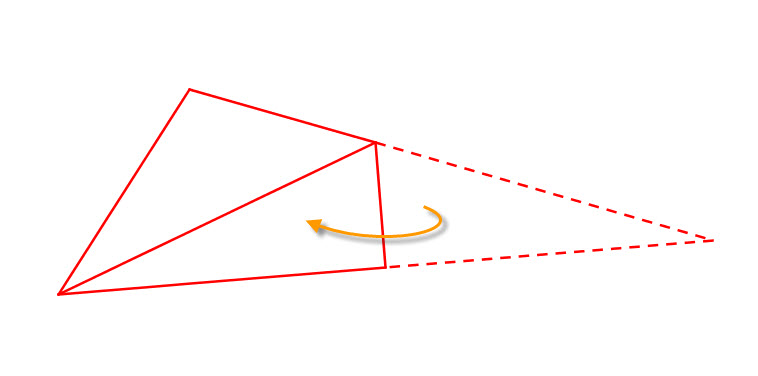Choose one triangle. Label the vertices A, B and C. Label the point of intersection of the perpenticular bisectors O. Then measure the distances AO, BO and CO. What do you observe? Take notes in your portfolio! Definition: A perpendicular bisector is a line that forms a right angle with one of the triangle's sides and intersects that side at its midpoint. The three perpendicular bisectors meet in a single point, called the triangle's circumcenter. The circumcenter of a triangle is the point of concurrency of the perpendicular bisectors of the sides of a triangle. This point is the center of the circumcircle, i.e. the circle passing through all three vertices. In general, 'to bisect' something means to cut it into two equal parts. The 'bisector' is the thing doing the cutting. With a perpendicular bisector, the bisector always crosses the line segment at right angles (90°). Links: The following links may be helpful for constructions only using a compass and straightedge: activity, take notes in your portfolio, repetition
 1.8 The circumcenter's positionThe circumcenter's position depends on the type of triangle: If and only if a triangle is acute (all angles smaller than a right angle), the circumcenter lies inside the triangle If and only if it is obtuse (has one angle bigger than a right angle), the circumcenter lies outside If and only if it is a right triangle, the circumcenter lies on one of its sides (namely, the hypotenuse). This is one form of Thales' theorem. Click here to "check" these facts! You will be able to change the size of the triangle by grabbing vertex A, B or C.
 1.9 Angle bisectors & the incenterDefinition: An angle bisector divides the angle into two angles with equal measures. The angle bisectors of the angles of a triangle are concurrent in one single point called the incenter of the triangle, which is the center of the incircle. In geometry, the incircle or inscribed circle of a triangle is the largest circle contained in the triangle, i.e. it touches (is tangen to) the three sides. Links: The following links may be helpful for constructions only using a compass and straightedge: take notes in your portfolio
 1.10 Logical ReasoningCloze Text: To check your knowledge do the following cloze text: Logical Reasoning